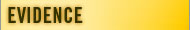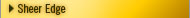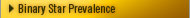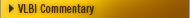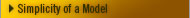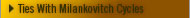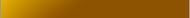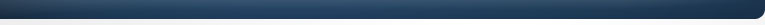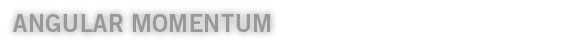The most widely accepted explanation for how the solar system formed is the Nebular Hypothesis. In this theory, the whole Solar System starts as a large cloud of gas that contracts under self-gravity. Conservation of angular momentum requires that a rotating disk form with a large concentration at the center (the proto-Sun). The centifugal force balances the gravitational forces and the disk coagulates into planets. Most people accept this theory, but there is one big unresolved problem - the angular momentum should be proportional to mass (as every physics student knows) but it's not in our solar system. The angular momentum issue is a well documented problem that has baffled solar system formation theorists for many years.The Sun contains 99.9 % mass, but only 1% of the total Angular Momentum. Most of the remainder is typically associated with the Jovian Planets. Theoretical Physicists developing Formation Theories are thwarted by this anomalous distribution. The Binary Model provides allocations of Angular Momentum to Mass for Planets and Stars in line with common expectations. An object in Rotational motion has an Angular Momentum L equal to its moment of inertia S (mi r2i) times its angular velocity w. If there is no external Torque, then L = Constant (Conservation of Angular Momentum). Our proof here is rather compelling. We first looked at the angular momentum distribution charts (see here 60 percent of angular momentum lies with Jupiter). We then ran the formulas ourselves with existing inputs to make sure the textbook data was correct. Everything checked out.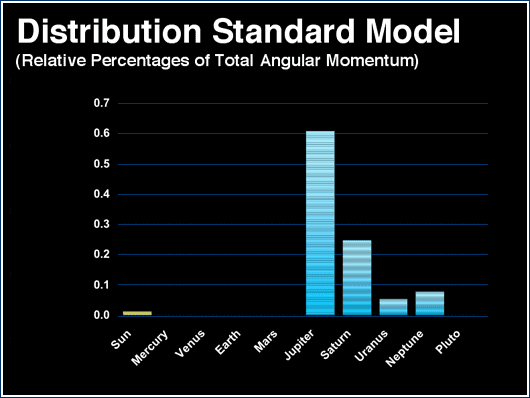Next, is the same chart in an "Angular Momentum to Mass ratio" formula. You can see all the bodies in our solar system have ratios in line with their mass except for the Sun.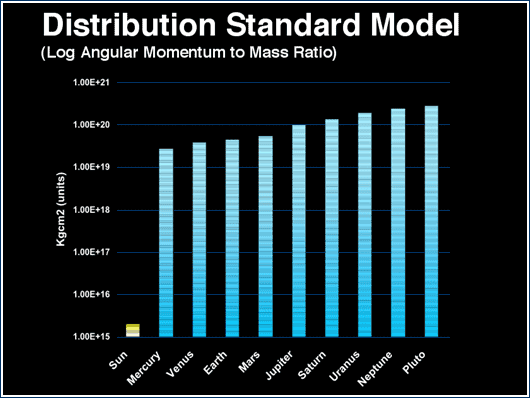We then added one input into the existing formula: we assumed the Sun was moving in a binary orbit with a period of 24,000 years.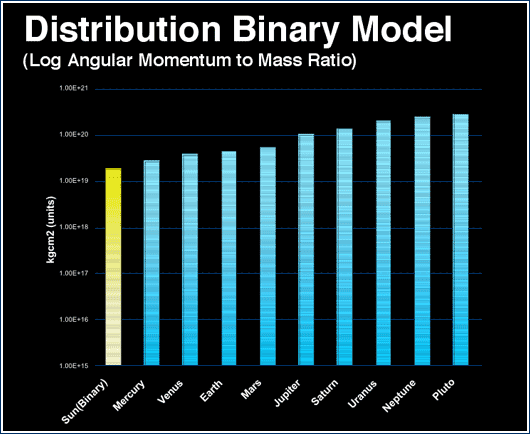As you can see, the Sun came right into line. This indicates the Sun may indeed have it's proper angular momentum (proportional to it's mass) providing another indication our sun is part of a binary or multiple star system.